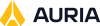# Figure of Merit

When the schedule deconflictor is run using certain algorithms, several different deconflicted solutions may be generated. STK Scheduler uses a Figure of Merit (FOM) calculation to determine the most preferred schedule solution from the family of deconflicted solutions.

The FOM equation is used to score all of the available deconflicted solutions to determine which one is the best for the user. The solution with the greatest FOM value is the one that is presented.

The FOM calculation features a series of terms, each designed to highlight a particular trait of a deconflicted solution. Each term is multiplied by a user-defined constant, the K value, to give weight to that particular trait. You may alter the default K values fir these constants to produce a deconflicted solution that matches his needs. Changing on K values relative to the others makes that attribute more or less important than the others, depending on whether the K value is larger or smaller, respectively, than the other K values.

Therefore, the only thing You must be aware of, when setting the FOM weights, is the ratios of the various K values. The domain of K values may be set at the user's discretion. For instance, if the K values are set between 0 and 100, Scheduler will present the same solution as if all of the K values are divided by 100, giving a domain of 0 to 1.

The Figure of Merit calculation is not used with the One-Pass deconfliction algorithm.

## The FOM FormulaWhere:

• Weight is the weight given to a task. The weight is calculated by the user-defined task priority of each task.

• Kassign is the weight given to tasks that are assigned. The value may be defined on the FOM Properties Tab of the Schedule Properties Form.

• Duration/DesiredDuration is a term that is meant to represent a task's assigned state. The value will be zero if the task is not assigned.

• Kdur is the weight given to tasks with longer duration. The value may be defined on the FOM Properties Tab of the Schedule Properties Form.

• Duration is the assigned duration of the task.

• KPP is the weight given to the Possibility Priority. The value may be defined on the FOM Properties Tab of the Schedule Properties Form. The value used for KPP is the sum of the KPP and KPN values in the tab.

• Possibility Priority is the average priority of all of the resource of a timeslot's possibility.

• KPN and the PositionRanking term do not contribute to the FOM in recent software versions.

• Kearly is the weight given to tasks that are assigned as early as possible. The value may be defined on the FOM Properties Tab of the Schedule Properties Form.

• EarlyBonus is a function that increases as the task assigned start time approaches its earliest.

• KmaxDur is the weight given to tasks that are assigned longer duration. The value may be defined on the FOM Properties Tab of the Schedule Properties Form.

• MaxBonus is a function that increases as the task's assigned duration approaches its maximum.

• KPT is the weight given to tasks that are assigned close to their preferred start time. The value may be defined on the FOM Properties Tab of the Schedule Properties Form.

• PreferredTimeBonus is a function that increases as the task start time approaches the preferred start time.

## How FOM is Used

When the Schedule De-conflictor is run using certain algorithms, a set of deconflicted solutions is generated. STK Scheduler will calculate a FOM value for each of these solutions. The solution with the highest FOM is then selected as the solution to be shown to the user.